# Python Tutorial – 13 – Prime Number Program

Learn how to generate prime numbers.

## 15 thoughts on “Python Tutorial – 13 – Prime Number Program”

1.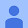Sreeja Bharathan says:

If user give 2

2.Pratik Matkar says:
3.Zacharias Papadopoulos says:

Man can you make a program that you put in the rank of a prime number (Ex. the 2nd prime number) and it gives you 3?(3 is the second prime)

4.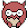Maximilian says:

luufa duufa duufa difoo! Bing bang billababingbong!

5.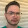0000 0000 says:

This system is flawed at 10:20 you can see that it says 4 is prime. It isn't.

6.Frag. says:

line 7, in <module>
for factor in range(2, number ):
TypeError: 'str' object cannot be interpreted as an integer

7.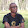Aaron Berci says:

WHat's that IDE ??

8.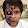Dhruvo DZ says:

r u using sublime?

9.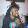Prella Hollie says:

What if your trying to find the prime factors of a users input?

10.thekoolaidman111 says:

totally farts at 4:18

11.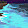Aminata Sankoh says:

The Main Program

Write a program that allows the user to select from one of the following tasks. After the task
runs, the user should be able to select another task. There should also be an option to end the
program when they are done.

Simple Divisibility Test

Determine if a number, n, is evenly divisible by a number, m. Both n and m are entered by the
user. The program should prompt the user for both numbers and then report if n is evenly
divisible by m.

Prime Test

Report if a user entered integer is prime. The user should be prompted for the number. When
displaying the result to the display, be sure to be descriptive so that the program is user friendly.

Factors

This task should ask the user to enter a positive integer, and report all positive integers that are a
factor of the user entered number. If zero or a negative number is entered, the user should be
informed that the number is not positive and be prompted to repeatedly enter an integer until a
positive integer is entered.

i have this project and am not a tech or science major so i am totally confused with this project. please help me

12.Gavin dmello says:

I had to cast the input method to an integer. Its maybe because I'm working on an older version of python

13.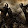bignate says:

I found a flaw when you input 4 it says that is prime but it is actually a composite number at 10:19. This is because the square root of 4 is 2 so it skips over 2 and marks it prime, the initial state of it.

14.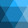greatsea says:

I evaluated the following in IDLE with a discrepancy that you might be able to explain to me:

>>>def is_prime(n):
for i in range(3, n):
if n % i == 0:
return False
return n

>>>filter(is_prime, range(3,50))
[3, 4, 5, 7, 11, 13, 17, 19, 23, 29, 31, 37, 41, 43, 47]

What the heck is the number '4' doing in there?

15.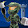DLC ENERGY says:

I made it on python 3.3 but i had to change it. Here's what i did:

def prime(number):
if number<2: return False
for factor in range(2, int(number**.5)+1):
if number%factor==0: return False
return True

print(prime(1)) # This will print(False)

1.Return False if number less than 2
2.Return False if number%factor==0 for factor in range 2 and sqrt(number)
(If sqrt(number) is a fraction round it up)
3.Return True if you reach the end